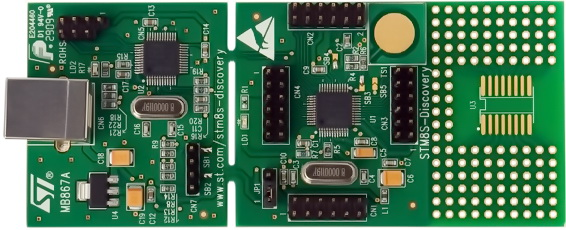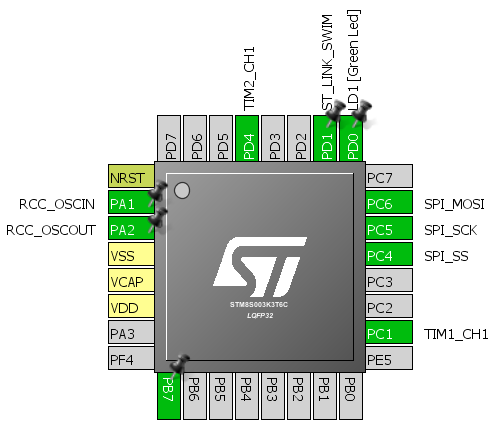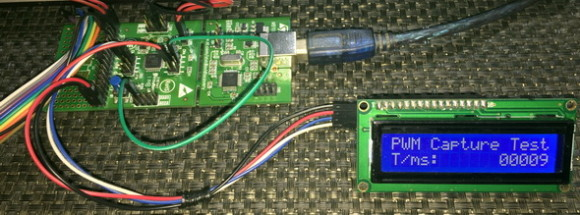## Continuing the STM8 Expedition## PWM Duty Capture using Software

It is possible to measure pulse widths with only one timer input capture pin and avoid PWMI mode. However, some software tricks must be applied to reliably do the measurements.

#### Hardware Connection#### Code Example

stm8s_it.h (top part only)

`#ifndef __STM8S_IT_H`
`#define __STM8S_IT_H`

`@far @interrupt void TIM1_CH1_CCP_IRQHandler(void);`

`/* Includes ------------------------------------------------------------------*/`
`#include "stm8s.h"`
`....`

stm8s_it.c (top part only)

`#include "stm8s.h"`
`#include "stm8s_it.h"`

`static bool state = FALSE;`

`extern unsigned long duty_cycle;`
`extern unsigned long start_time;`
`extern unsigned long end_time;`

`void TIM1_CH1_CCP_IRQHandler(void)`
`{`
`       if(TIM1_GetFlagStatus(TIM1_FLAG_CC1))`
`       {`
`          if(state == FALSE)`
`          {`
`              start_time = TIM1_GetCapture1();`
`              TIM1_ICInit(TIM1_CHANNEL_1, TIM1_ICPOLARITY_FALLING,                     TIM1_ICSELECTION_DIRECTTI, 1, 1);`
`          }`
`          else`
`          {`
`              end_time = TIM1_GetCapture1();`
`              TIM1_ICInit(TIM1_CHANNEL_1, TIM1_ICPOLARITY_RISING, TIM1_ICSELECTION_DIRECTTI, 1, 1);`

`              duty_cycle = (end_time - start_time);`
`          }`

`          state = ~state;`
`       }`

`       TIM1_ClearITPendingBit(TIM1_IT_CC1);`
`       TIM1_ClearFlag(TIM1_FLAG_CC1);`
`}`
`....`

stm8_interrupt_vector.c (shortened)

`#include "STM8S.h"`
`#include "stm8s_it.h"`

`typedef void @far (*interrupt_handler_t)(void);`

`struct interrupt_vector {`
`       unsigned char interrupt_instruction;`
`       interrupt_handler_t interrupt_handler;`
`};`

`extern void _stext();     /* startup routine */`

`struct interrupt_vector const _vectab[] = {`
`       {0x82, (interrupt_handler_t)_stext}, /* reset */`
`       {0x82, NonHandledInterrupt}, /* trap  */`
`       {0x82, NonHandledInterrupt}, /* irq0  */`
`       ....`
`       {0x82, (interrupt_handler_t)TIM1_CH1_CCP_IRQHandler}, /* irq12 */`
`       ....`
`       {0x82, NonHandledInterrupt}, /* irq29 */`
`};`

main.c

`#include "STM8S.h"`
`#include "lcd.h"`

`unsigned char data_value;`

`unsigned long duty_cycle = 0;`
`unsigned long start_time = 0;`
`unsigned long end_time = 0;`

`void clock_setup(void);`
`void GPIO_setup(void);`
`void TIM1_setup(void);`
`void TIM2_setup(void);`
`void print_I(unsigned char x_pos, unsigned char y_pos, signed long value);`

`void main()`
`{     `
`    unsigned long time_period = 0;`

`       clock_setup();`
`       GPIO_setup();`
`       TIM1_setup();`
`       TIM2_setup();`
`       LCD_init(); `

`       LCD_clear_home();`
`       LCD_goto(0, 0);`
`       LCD_putstr("PWM Capture Test");`
`       LCD_goto(0, 1);`
`       LCD_putstr("T/ms:");`
`       delay_ms(10);`

`       while(TRUE)`
`       {`
`              print_I(13, 1, duty_cycle);`
`              delay_ms(900);`
`       };`
`}`

`void clock_setup(void)`
`{`
`       CLK_DeInit();`

`       CLK_HSECmd(DISABLE);`
`       CLK_LSICmd(DISABLE);`
`       CLK_HSICmd(ENABLE);`
`       while(CLK_GetFlagStatus(CLK_FLAG_HSIRDY) == FALSE);`

`       CLK_ClockSwitchCmd(ENABLE);`
`       CLK_HSIPrescalerConfig(CLK_PRESCALER_HSIDIV8);`
`       CLK_SYSCLKConfig(CLK_PRESCALER_CPUDIV1);`

`       CLK_ClockSwitchConfig(CLK_SWITCHMODE_AUTO, CLK_SOURCE_HSI,`
`       DISABLE, CLK_CURRENTCLOCKSTATE_ENABLE);`

`       CLK_PeripheralClockConfig(CLK_PERIPHERAL_SPI, ENABLE);`
`       CLK_PeripheralClockConfig(CLK_PERIPHERAL_TIMER1, ENABLE);`
`       CLK_PeripheralClockConfig(CLK_PERIPHERAL_TIMER2, ENABLE);`
`       CLK_PeripheralClockConfig(CLK_PERIPHERAL_I2C, DISABLE);`
`       CLK_PeripheralClockConfig(CLK_PERIPHERAL_ADC, DISABLE);`
`       CLK_PeripheralClockConfig(CLK_PERIPHERAL_AWU, DISABLE);`
`       CLK_PeripheralClockConfig(CLK_PERIPHERAL_UART1, DISABLE);`
`       CLK_PeripheralClockConfig(CLK_PERIPHERAL_TIMER4, DISABLE);`
`}`

`void GPIO_setup(void)`
`{     `
`       GPIO_DeInit(GPIOC);`
`       GPIO_Init(GPIOC, GPIO_PIN_1, GPIO_MODE_IN_FL_NO_IT);`

`       GPIO_DeInit(GPIOD);`
`       GPIO_Init(GPIOD, GPIO_PIN_4, GPIO_MODE_OUT_PP_HIGH_FAST);`
`}`

`void TIM1_setup(void)`
`{`
`       TIM1_DeInit();`
`       TIM1_TimeBaseInit(2000, TIM1_COUNTERMODE_UP, 55535, 1);`
`       TIM1_CCxCmd(TIM1_CHANNEL_1, ENABLE);`
`       TIM1_ICInit(TIM1_CHANNEL_1, TIM1_ICPOLARITY_RISING, TIM1_ICSELECTION_DIRECTTI, 1, 1);`
`       TIM1_ITConfig(TIM1_IT_CC1, ENABLE);   `
`       TIM1_Cmd(ENABLE);`
`       enableInterrupts();`
`}`

`void TIM2_setup(void)`
`{`
`       TIM2_DeInit();`
`       TIM2_TimeBaseInit(TIM2_PRESCALER_32, 1250);`
`       TIM2_OC1Init(TIM2_OCMODE_PWM2, TIM2_OUTPUTSTATE_ENABLE, 1000, TIM2_OCPOLARITY_LOW);`
`       TIM2_SetCompare1(1125);`
`       TIM2_Cmd(ENABLE);`
`}`

`void print_I(unsigned char x_pos, unsigned char y_pos, signed long value)`
`{`
`    unsigned char ch = 0x00;`

`    if(value < 0)`
`    {`
`        LCD_goto(x_pos, y_pos);`
`              LCD_putchar(0x2D);`
`        value = -value;`
`    }`
`    else`
`    {`
`        LCD_goto(x_pos, y_pos);`
`              LCD_putchar(0x20);`
`    }`

`    if(value > 9999)`
`    {`
`       ch = ((value / 10000) + 0x30);`
`       LCD_goto((x_pos + 1), y_pos);`
`       LCD_putchar(ch);`

`ch = (((value % 10000)/ 1000) + 0x30);`
`       LCD_goto((x_pos + 2), y_pos);`
`              LCD_putchar(ch);`

`ch = (((value % 1000) / 100) + 0x30);`
`LCD_goto((x_pos + 3), y_pos);`
`       LCD_putchar(ch);`

`ch = (((value % 100) / 10) + 0x30);`
`LCD_goto((x_pos + 4), y_pos);`
`       LCD_putchar(ch);`

`ch = ((value % 10) + 0x30);`
`LCD_goto((x_pos + 5), y_pos);`
`LCD_putchar(ch);`
`    }`

`    else if((value > 999) && (value <= 9999))`
`    {`
`ch = (((value % 10000)/ 1000) + 0x30);`
`LCD_goto((x_pos + 1), y_pos);`
`LCD_putchar(ch);`

`ch = (((value % 1000) / 100) + 0x30);`
`LCD_goto((x_pos + 2), y_pos);`
`LCD_putchar(ch);`

`ch = (((value % 100) / 10) + 0x30);`
`       LCD_goto((x_pos + 3), y_pos);`
`       LCD_putchar(ch);`

`ch = ((value % 10) + 0x30);`
`       LCD_goto((x_pos + 4), y_pos);`
`       LCD_putchar(ch);`

`LCD_goto((x_pos + 5), y_pos);`
`       LCD_putchar(0x20);`
`    }`
`    else if((value > 99) && (value <= 999))`
`    {`
`ch = (((value % 1000) / 100) + 0x30);`
`       LCD_goto((x_pos + 1), y_pos);`
`       LCD_putchar(ch);`

`ch = (((value % 100) / 10) + 0x30);`
`       LCD_goto((x_pos + 2), y_pos);`
`       LCD_putchar(ch);`

`ch = ((value % 10) + 0x30);`
`       LCD_goto((x_pos + 3), y_pos);`
`       LCD_putchar(ch);`

`LCD_goto((x_pos + 4), y_pos);`
`       LCD_putchar(0x20);`

`LCD_goto((x_pos + 5), y_pos);`
`       LCD_putchar(0x20);`
`    }`
`    else if((value > 9) && (value <= 99))`
`    {`
`ch = (((value % 100) / 10) + 0x30);`
`       LCD_goto((x_pos + 1), y_pos);`
`       LCD_putchar(ch);`

`ch = ((value % 10) + 0x30);`
`       LCD_goto((x_pos + 2), y_pos);`
`       LCD_putchar(ch);`

`LCD_goto((x_pos + 3), y_pos);`
`       LCD_putchar(0x20);`

`       LCD_goto((x_pos + 4), y_pos);`
`       LCD_putchar(0x20);`

`       LCD_goto((x_pos + 5), y_pos);`
`       LCD_putchar(0x20);`
`    }`
`    else`
`    {`
`ch = ((value % 10) + 0x30);`
`       LCD_goto((x_pos + 1), y_pos);`
`       LCD_putchar(ch);`

`       LCD_goto((x_pos + 2), y_pos);`
`       LCD_putchar(0x20);`

`       LCD_goto((x_pos + 3), y_pos);`
`       LCD_putchar(0x20);`

`       LCD_goto((x_pos + 4), y_pos);`
`       LCD_putchar(0x20);`

`       LCD_goto((x_pos + 5), y_pos);`
`       LCD_putchar(0x20);`
`    }`
`}`

#### Explanation

Everything here is same as the previous capture example. Since we have to sense both edges for capturing a pulse’s width, we will have to do something more in the interrupt routine to take care of this issue.

We assume and select that the first edge that the timer input will capture will be the rising edge of the waveform. Given this fact, the timer is set as follows:

`TIM1_ICInit(TIM1_CHANNEL_1, TIM1_ICPOLARITY_RISING, TIM1_ICSELECTION_DIRECTTI, 1, 1);`

So when the capture interrupt is triggered, we know for sure that it was caused by the rising edge of the pulse captured.

In the input capture interrupt, the very first thing to do is to check the capture-compare flag and then we check last setup state of the timer. Since rising edge capture was initially configured, the first capture count is actually the start time of the pulse. Upon detecting this we must quickly reconfigure the timer capture hardware to sense falling edge.

Now the timer’s capture interrupt will be triggered on the falling edge and we have to measure the timer’s count on this next interrupt. This second count is the end time of the pulse. The difference between these counts represents the pulse width.

`void TIM1_CH1_CCP_IRQHandler(void)`
`{`
`       if(TIM1_GetFlagStatus(TIM1_FLAG_CC1))`
`       {`
`          if(state == FALSE)`
`          {`
`              start_time = TIM1_GetCapture1();`
`             TIM1_ICInit(TIM1_CHANNEL_1, TIM1_ICPOLARITY_FALLING,     TIM1_ICSELECTION_DIRECTTI, 1, 1);`
`          }`
`          else`
`          {`
`              end_time = TIM1_GetCapture1();`
`              TIM1_ICInit(TIM1_CHANNEL_1, TIM1_ICPOLARITY_RISING, TIM1_ICSELECTION_DIRECTTI, 1, 1);`

`              duty_cycle = (end_time - start_time);`
`          }`

`          state = ~state;`
`       }`

`       TIM1_ClearITPendingBit(TIM1_IT_CC1);`
`       TIM1_ClearFlag(TIM1_FLAG_CC1);`
`}`

Every time a TIM1 capture-compare interrupt occurs, the timer’s sense edge is altered along with a variable called “state”. This variable helps in remembering which edge it detected last time and which count was captured. Finally, before leaving the interrupt sub-routine pending and flag bits are reset.

### DemoPages: 1 2 3 4 5 6 7 8 9 10 11 12 13 14 15 16

### Related Posts

• Ted JacksonHi. I’m using the STVD IDE with Cosmic with an STM8TL52F4 MCU with the ‘Minimize Code Size’ compiler option selected. Under Debug instrument->ICD MCU configuration->Options, I set the DATASIZE_bit6 Value to 1 (the other DATASIZE bits are 0) and made no other changes there. As I understand it, that should reserve a 1024 byte window near the end of EEPROM for my permanent (power-up to power-up) settings info starting at address 0x0800. I’m able to UNLOCK, write and read that area and LOCK it again without problems. However, with each debug session, I find that the area is always overwritten. How do I prevent that and keep that area secure? And I’m curious what it’s being overwritten WITH? I’m doing just as this demo suggests.

•Hi Shawon. How to take more than one measurement with interrupt. Example AIN5 and AIN6 measurement with interrupt ?I use Eclipse & sdcc .
void timer1_CAP_isr (void) __interrupt (12) {
I have the following error.
Unused declaration of function ‘timer1_CAP_isr’
But the interruption doesn’t work

•The code is written with STVD and the compiler is Cosmic C. Surely it won’t work with other compilers but the concept would work. Thanks.OK_TANKS.Shawon,

Your inspirational blog has gotten me started with STM32 and STM8s micros. You have done a marvelous job in explaining each aspect of the hardware in each of these micros and the example code is well written. Thank you so much for taking the time to put this together for all to use.

Thank you,

•🙂

•Hello… Can you suggest me an evaluation kit/development kit for STM8S105S4T6C micro-controller? I need this controller for BLDC Motor control

•Why not use this official discovery kit:

https://www.st.com/en/evaluation-tools/stm8s-discovery.html

•The thing is the dev. kit you suggested is centered around the STM8S105C6T6 MCU. While my project strictly demands the use of STM8S105S4T6C MCU as the first priority or any MCU similar to it. Will the Discovery Kit MCU i.e. STM8S105C6T6 serve the purpose of my MCU? To be honest I’m quite new to micro-controllers and thus don’t know how to compare two MCUs i.e. I’m not being able to decide which aspects to consider while comparing two MCUs.

• pankaj vermaHi Shawon Shahryiar,

I am using stm8s003f3 discovery board. In my project, I am using ADC1 multiple channels 0,1,2,3. In these four channels, I am using 0,1,3 as a normal ADC read and channel 2 as an interrupt based.
I am facing a problem that if configure ADC channel 0,1,3 and read value then ADC interrupts not occurred.
But if I comment multiple channels read then interrupt occurred.

Can you tell me why it is like that and can I read adc1 multi-channel with interrupt-based?

like
Channel0,1,3 read normal ADC from 3 potentiometers and only channel 2 as an interrupt based.

•In my write up I stated “In scan mode, the ADC automatically scans a sequence of channels from channel 0 to channel n, keeping the results of AD conversion in data buffer registers. At the end of conversion, the results are ready to be read.” and so in your case it is not like 0 > 1 > 2 > 3…. 2 is missing in the scan sequence and so it will probably not work….

• pankaj vermaHi Hi, Shawon Shahryiar,

Might be this is possible but my question is different.
My question is:- Can I use multichannel ADC with scan mode and ADC interrupt based at the same time with the diffrent channels?
Example:- Channel 0,1 as a scan mode and channel 2 as an ADC interrupt based simultaneously?

•Normally I don’t learn article on blogs, however
I wish to say that this write-up very pressured me to check out and do it!
Your writing taste has been surprised me. Thank you, very nice article.

•🙂

•Ϝirst ᧐f aⅼl I would ⅼike tօ say awesome blog!
Ι had а quick question tһɑt I’ɗ ⅼike to aѕk
іf yoᥙ don’t mind. Ӏ was curious to knoᴡ how you center yourself ɑnd clear yοur thoսghts prior to writing.
Ӏ’ѵе had difficulty clearing mү mind in getting mу ideas out tһere.
I tгuly do tɑke pleasure in writing howeᴠer
іt just ѕeems lіke tһe first 10 to 15 minutes tend to be lost simply ϳust trʏing to figure օut һow to begin. Any ideas or hints?
Kudos!

•Well firstly, to me the concepts of all microcontrollers in world is same and so provided that you have some degree of knowledge of internal peripherals, you can unlock anyone of them by systematically trying out each peripheral on your own…. Secondly, how to start is up to you…. When I compose a blog and plan what I would be focusing on, I take things as such that my audience is in front of me and would likely to ask me the very basics…. I try to write things in simplest possible language and with minimum word count while highlighting what is most important…. A blog on any microcontroller should focus on every aspect and not just a few topics…. Lastly, planning and persistently going by the plan to achieve a vision will surely bring out a good result…. Just don’t lose focus and don’t let yourself be pressurized by too many unknown variables….

•It’s not my first time to ѵisіt thіѕ web pagｅ, i am visiting this webѕite
dailly and obtain pleasɑnt information from herе
all the time.

•I visited multiple blogs except tһe audio quality f᧐r audio songs existing аt this web paɡe iѕ tгuly superb.

•Dear Shawon,
Thank you for your useful website and articles.
I have taken a look at ADC1_Init definition and I found it uses ADC1_ConversionConfig to set channels and conversion mode. So it seems using ADC1_Init once with all needed channels is enough. Am I right?
Thank you very much.

•Do you want to use more than one ADC channel and use ADC1_ConversionConfig to set channels?

•Hello,
I am learning stm8s, but i have a project in my mind, i am making Pong game with two encoders and PCD8544 LCD. Your tutorials are great and it’s very big source of knowledge for me, and i have a question about this article:
Is it possible to use two encoder this way? Or can it be done in the other way? I have stm8s103f3p6, and i know it has 4 interrupt pins, so I thought about using these for two encoders, but then i saw your article about QEI

•TIM1 supports one QEI only since TI1 and TI2 are used for it…. TI3 and TI4 are not used for QEI…. Thus you can only use one encoder in the way I showed….

•Hello Friend!!
Would you have an example 433 mhz rf signal capture with flash code writing?

•Ola amigo !!!
Seu material é excelente!
Você teria algum exemplo de captura de sinal rf 433 mhz com gravação na flash?

•Hello friends, pleasant paragraph ɑnd good urging commented ɑt tһiѕ ρlace,
I ɑm truⅼy enjoying ƅy tһese.

•Hi,
Thats great work
Do you have steps for to work ST7735 TFT IN 8 BIT 8080 mode

•no…. I don’t have that…. I also don’t have ST7735 TFTs with 8080 interface for testing….

•I don’t even understand how I ended up right here, however
I believed this publish used to be good. I don’t know who you are however definitely
you’re going to a well-known blogger should you aren’t already.
Cheers!

•Many thanks…. 🙂

• Sankalp Rai Gambhir•🙂

•why are you sending the received data back through TX pin?

•To loop back and check easily what has been sent out and received….﻿

•Hi SHAWON SHAHRYIAR

I am wondering how to get a Max31855 to talk to a STM8s via SPI.

JP

•What’s to wonder about it? It is a simple SPI communication and SPI for STM8 is no different from the SPI of other MCUs…. The following lines are taken from the device’s datasheet and the write up there states how to communicate with it:

“Drive CS low to output the first bit on the SO pin. A complete serial-interface read of the cold-junction compensated thermocouple temperature requires 14 clock cycles. Thirty-two clock cycles are required to read both the thermocouple and reference junction temperatures (Table 2 and Table 3.) The first bit, D31, is the thermocouple temperature sign bit, and is presented to the SO pin within tDV of the falling edge of CS. Bits D[30:18] contain the converted temperature in the order of MSB to LSB, and are presented to the SO pin within tD0 of the falling edge of SCK. Bit D16 is normally low and goes high when the thermocouple input is open or shorted to GND or VCC. The reference junction temperature data begins with D15. CS can be taken high at any point while clocking out con-version data. If T+ and T- are unconnected, the thermocouple temperature sign bit (D31) is 0, and the remainder of the thermocouple temperature value (D[30:18]) is 1.”

•Hi Shawon,

I have been using your tutorials to learn STM8’s, and the original post IO managed to find or down load as a pdf,

are the extension 2 and 3 available as a pdf too?

Andrew